## Interactive Figures

These are interactive figures that reproduce results from an open science article on an imaging denoising technique, anisotropic non-linear diffusion.

Results on this page are dynamically generated by your web browser, utilizing the same code and data presented in the article. Figure reproduction presets can be selected, which load the input image and set analysis parameters to the values used for a given article figure. It is also possible to modify parameters and see their effects. Or, run the algorithm on your own PNG image.

Input Image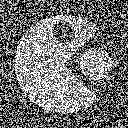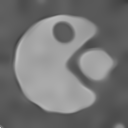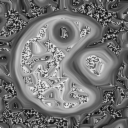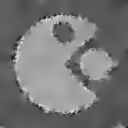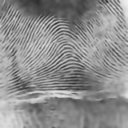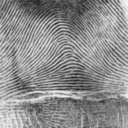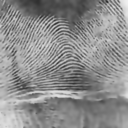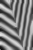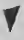Diffusion Time, $$T$$ 0.5         50 Lambda, $$\lambda$$ 0.5         0.0001 Weickert Diffusion Type Edge Enhancing Diffusion (EED) Conservative Edge Enhancing Diffusion (cEED) Coherence Enhancing Diffusion (CED) Conservative Coherence Enhancing Diffusion (cCED) Isotropic Noise Scale, $$\sigma$$ 0.5         5 Feature Scale, $$\rho$$ 2.0         6 Exponent, $$m$$ 1.0         6
Execution